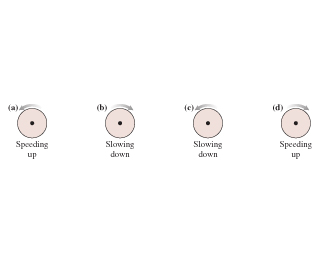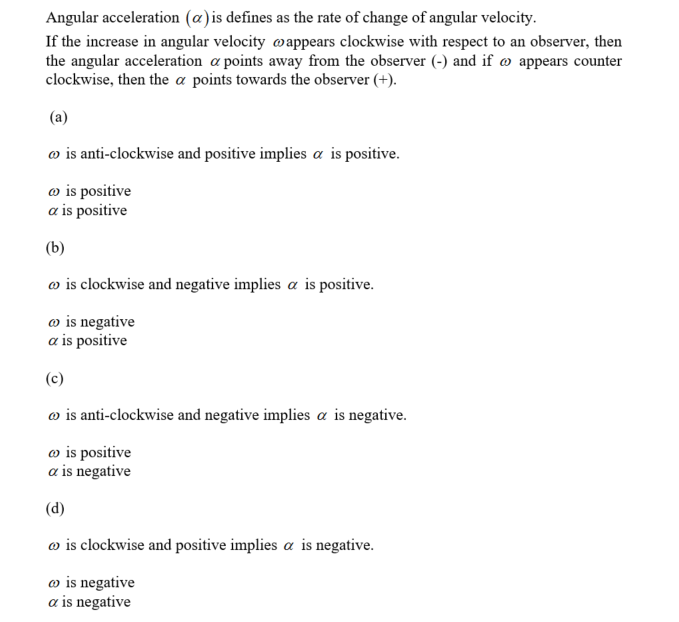# The figure(figure 1) shows four rotating wheels. for each. determine the signs (+ or -)of ω and α.

The figure(Figure 1) shows four rotating
wheels. For each, determine the signs (+ or -)of ω and
α. The figure(Figure 1) ω αAngular acceleration (a)is defines as the rate of change of angular velocity. If the increase in angular velocity ω appears clockwise with respect to an observer, then the angular acceleration α points away from. the observer (-) and if ω appears counter clockwise, then the α points towards the observer (+). ω is anti-clockwise and positive implies α is positive. ω is positive α is positive ais clockwise and negative implies α is positive ω is negative α is positive a) is anti-clockwise and negative implies α is negative. ω is positive α is negative is clockwise and positive implies α is negative. a, is negative α is negative